Crystal Systems

• Define crystal.
• Define crystal lattice.What are the different uses of lasers?

The development of modern lasers has opened many doors to both research and applications. A laser beam was used to measure the distance from the Earth to the moon. Lasers are important components of CD players. As the image above illustrates, lasers can provide precise focusing of beams to selectively destroy cancer cells in patients. The ability of a laser to focus precisely is due to high-quality crystals that help give rise to the laser beam. A variety of techniques are used to manufacture pure crystals for use in lasers.

Crystalline Solids

The majority of solids are crystalline in nature. A crystal is a substance in which the particles are arranged in an orderly, repeating, three-dimensional pattern. Particles of a solid crystal may be ions, atoms, or molecules, depending on the type of substance. The three-dimensional arrangement of a solid crystal is referred to as the crystal lattice . Different arrangements of the particles within a crystal cause them to adopt several different shapes.

Crystal Systems

Crystals are classified into general categories based on their shapes. A crystal is defined by its faces, which intersect with one another at specific angles, which are characteristic of the given substance. The seven crystal systems are shown below, along with an example of each. The edge lengths of a crystal are represented by the letters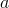,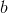, and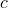. The angles at which the faces intersect are represented by the Greek letters $\alpha$, $\beta$, and $\gamma$. Each of the seven crystal systems differs in terms of the angles between the faces and in the number of edges of equal length on each face.

Seven Basic Crystal Systems and an Example of Each
Crystal System Diagram Example

Cubic

a = b = c; α = β = γ = 90°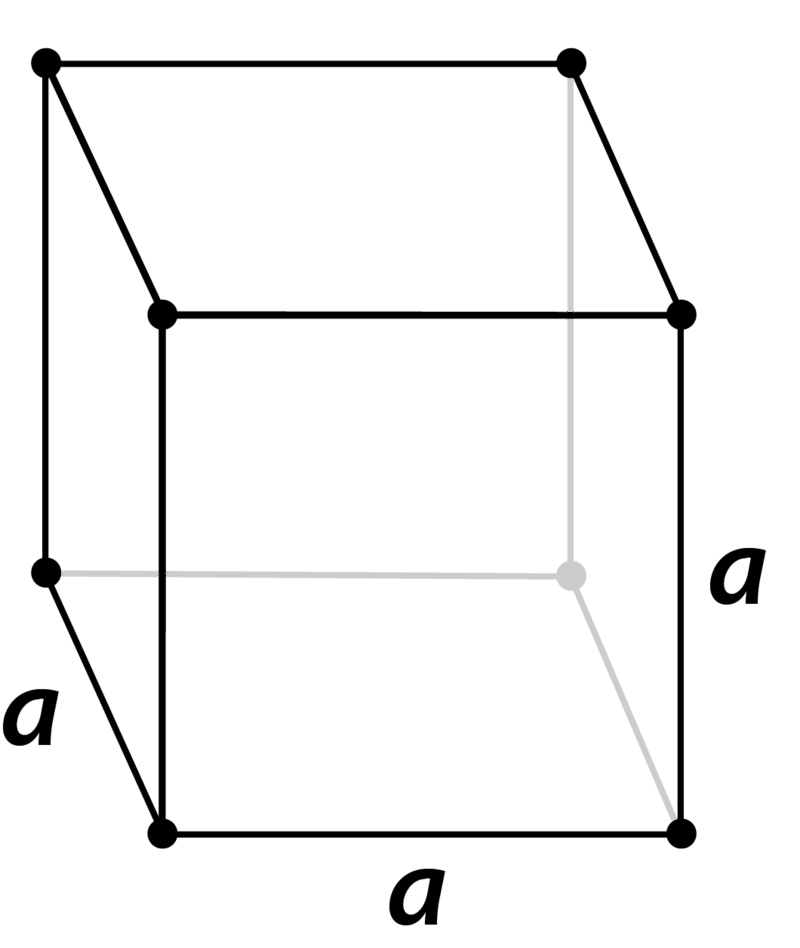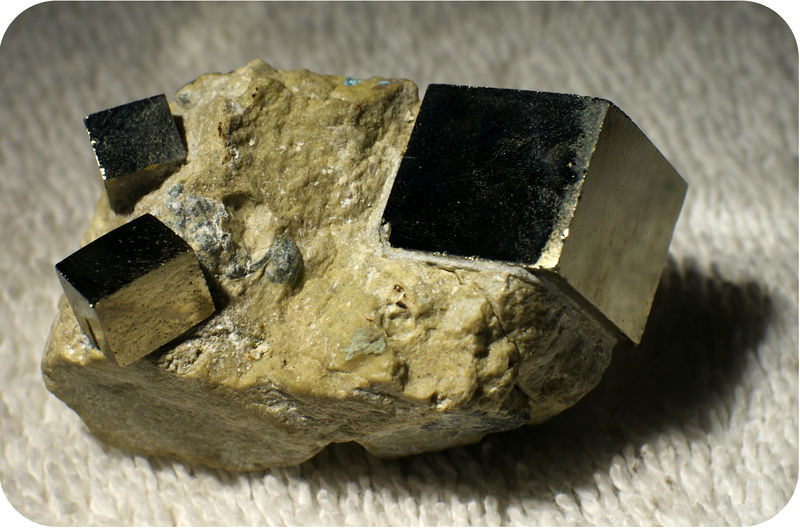Pyrite

Tetragonal

a = b ≠ c; α = β = γ = 90°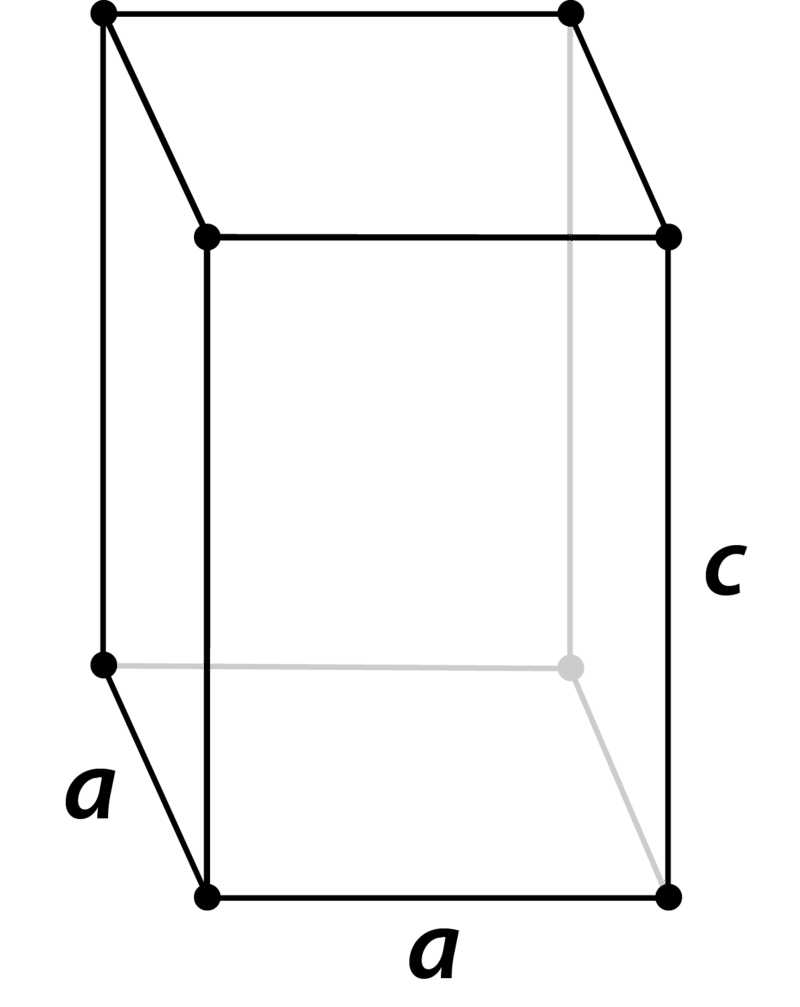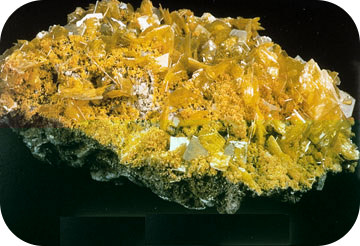Wulfenite

Orthorhombic

a ≠ b ≠ c; α = β = γ = 90°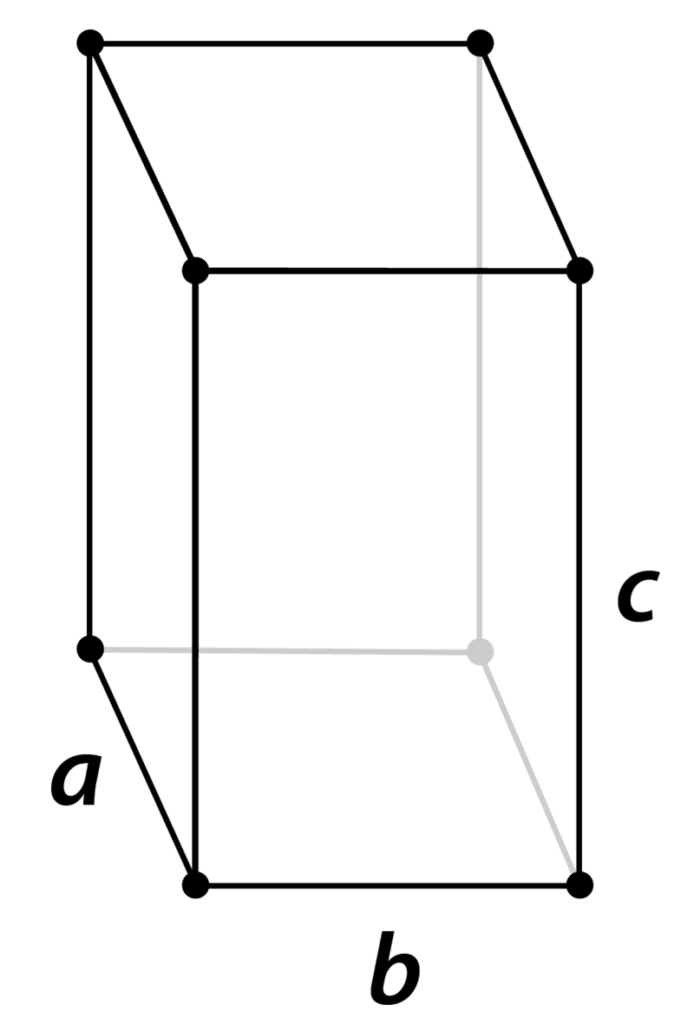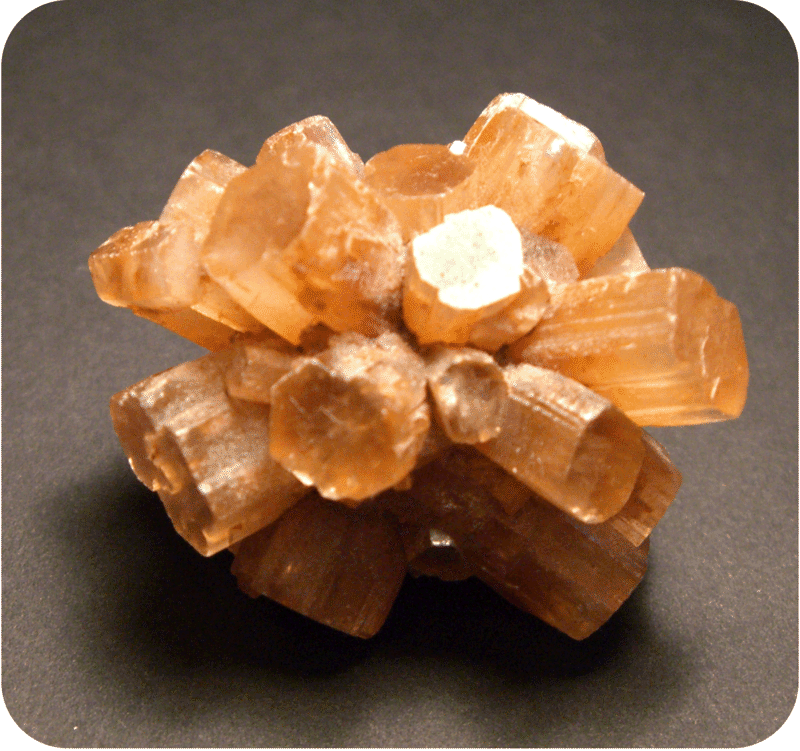Aragonite

Monoclinic

a ≠ b ≠ c; α ≠ 90° = β = γ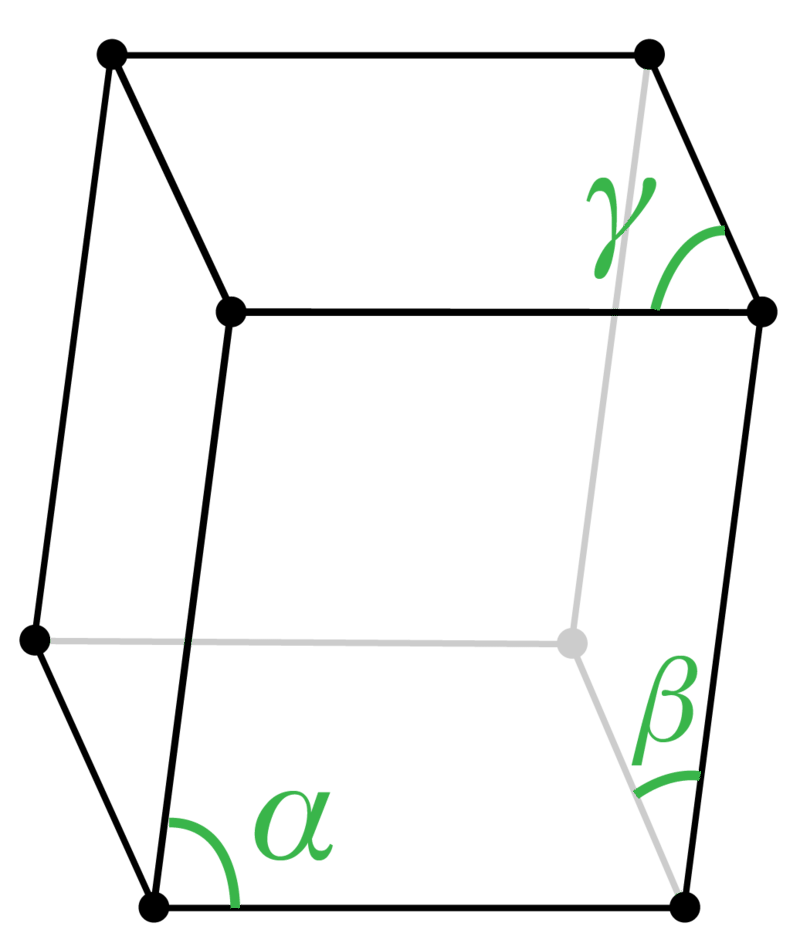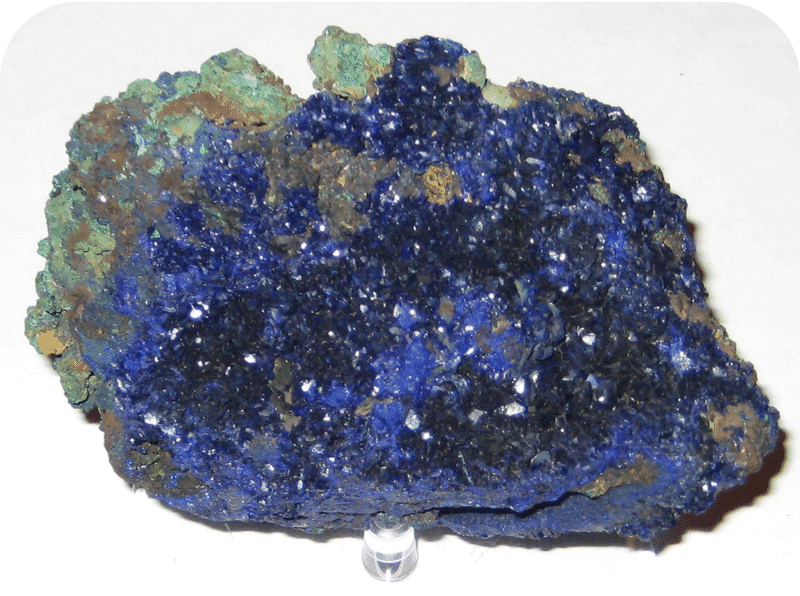Azurite

Rhombohedral

a = b = c; α = β = γ ≠ 90°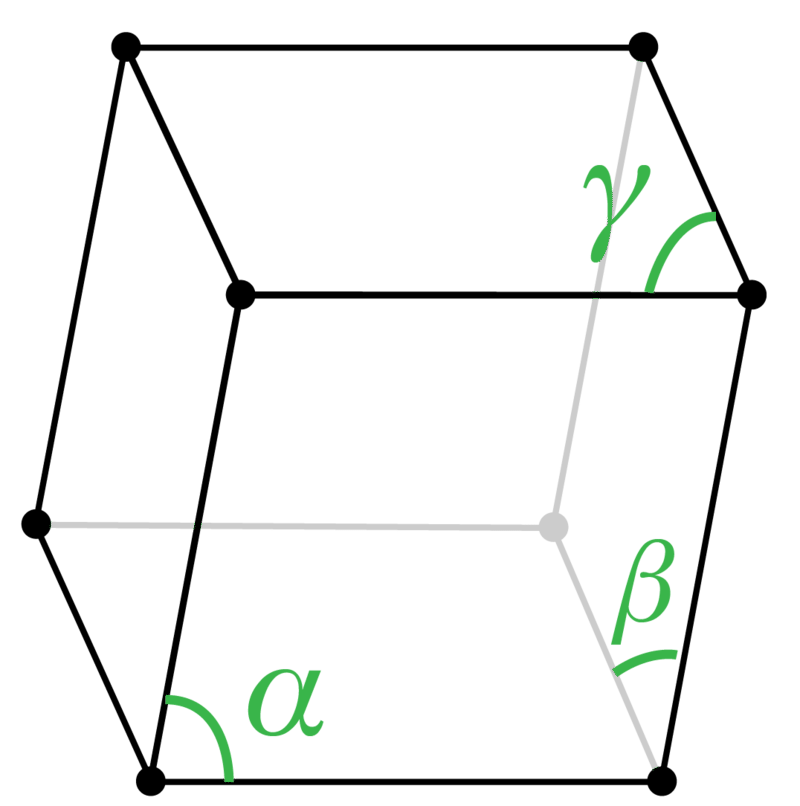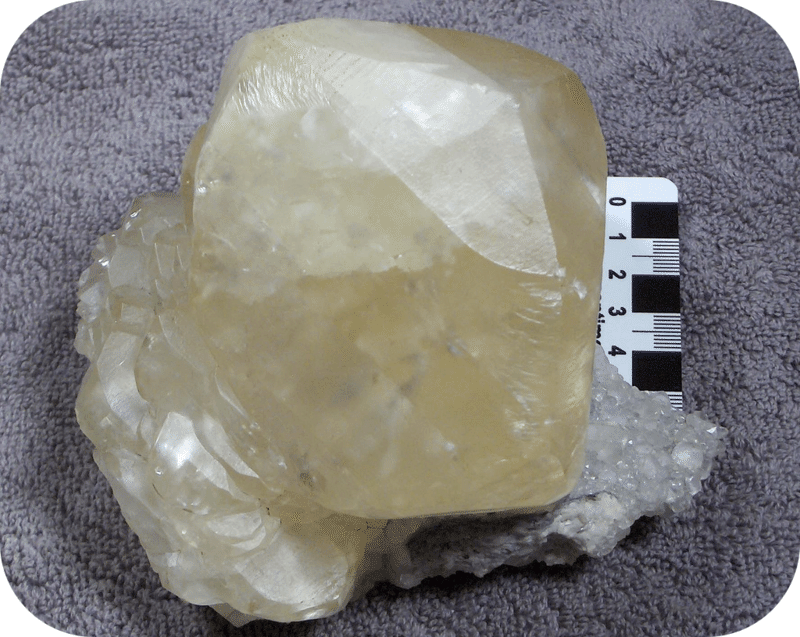Calcite

Triclinic

a ≠ b ≠ c; α ≠ β ≠ γ ≠ 90°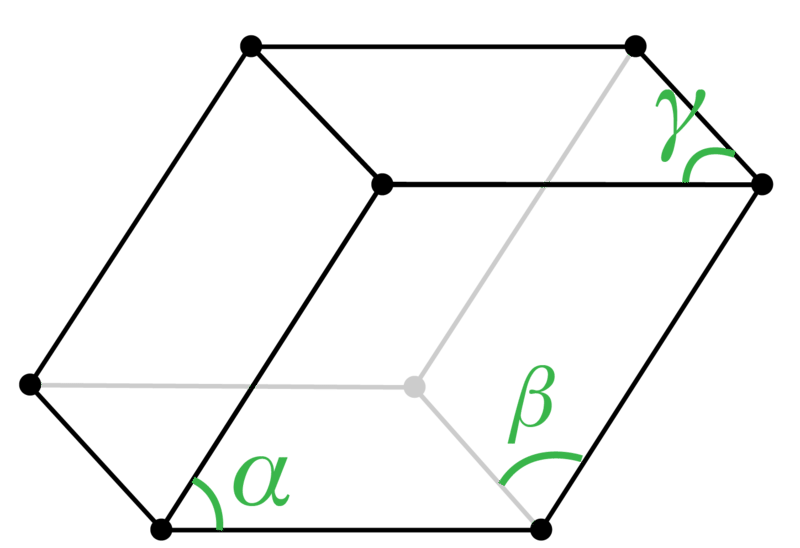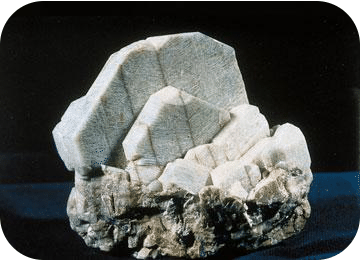Microcline

Hexagonal

a = b ≠ c; α = β = 90°, γ = 120°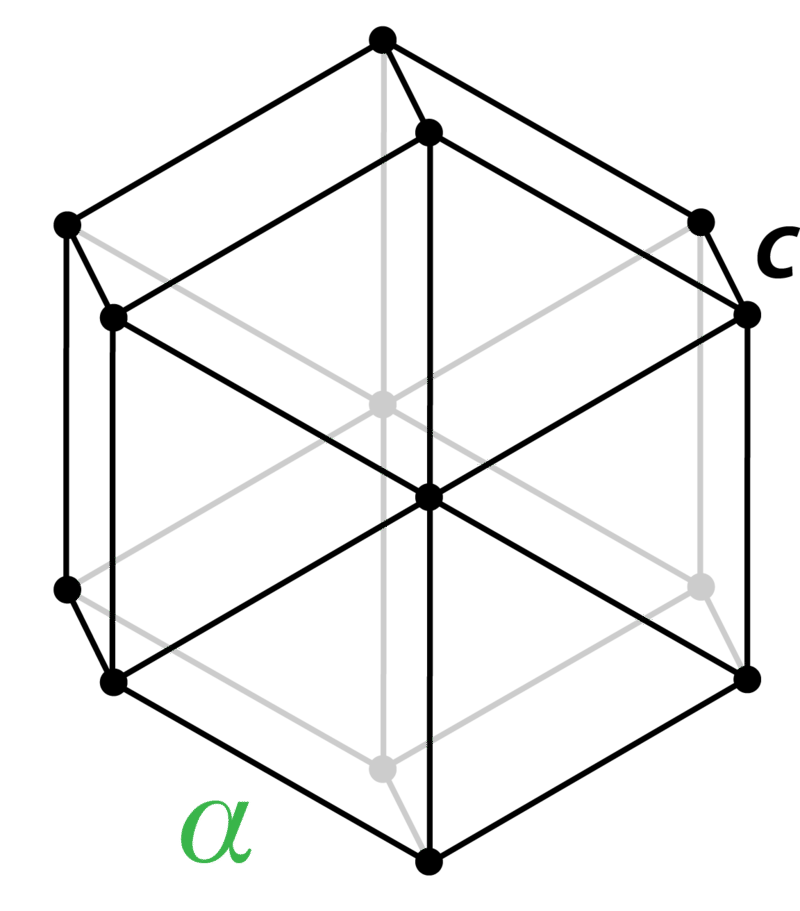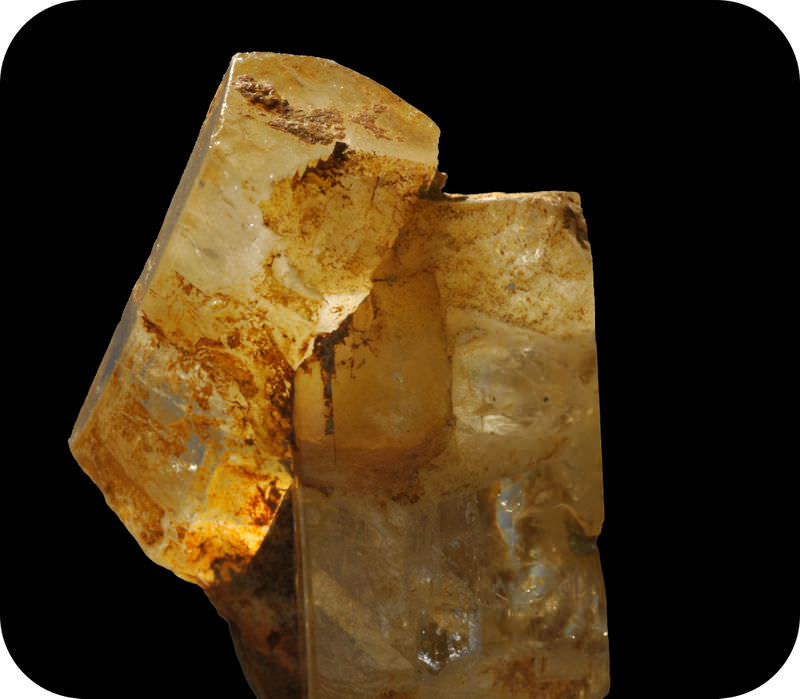Beryl

Unit Cells

Summary

• A crystal is a substance in which the particles are arranged in an orderly, repeating, three-dimensional pattern.
• The crystal lattice is the three-dimensional arrangement of a solid crystal.

Practice

Questions

http://www.bookrags.com/research/crystals-and-crystallography-woes-01/

1. What is crystallography?
2. What is a lattice?
3. What types of atomic bonds can exist in crystals?

Review

Questions

1. What is a crystal?
2. List the seven crystal systems.
• crystal: A substance in which the particles are arranged in an orderly, repeating, three-dimensional pattern.
• crystal lattice: The three-dimensional arrangement of a solid crystal.
• crystal system: A classification of crystals. There are seven systems.